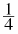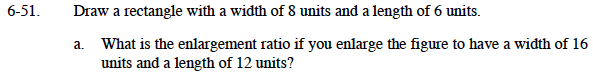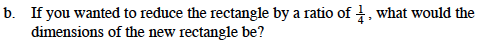### Home > MC1 > Chapter 6 > Lesson 6.2.1 > Problem6-51

6-51.
1. . Draw a rectangle with a width of 8 units and a length of 6 units. Homework Help ✎

1. What is the enlargement ratio if you enlarge the figure to have a width of 16 units and a length of 12 units?

2. If you wanted to reduce the rectangle by a ratio of, what would the dimensions of the new rectangle be?8(?) = 16
6(?) = 12What is one-fourth of 8? What is one-fourth of 6?

$\text{The rectangle would be 2 units by }1\frac{1}{2}\text{ units.}$